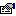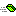## Int64Static

Provides methods for manipulating a 64-bit signed integer.

### Public:

#### Types:

NameDescriptionInt64 Represents a 64-bit signed integer.

#### Properties:

NameDescriptionMaxValue (get) Represents the largest possible value of an Int64.MinusOne (get) Returns an Int64 value represnting minus-one.MinValue (get) Represents the smallest possible value of an Int64.One (get) Returns an Int64 value representing one.Zero (get) Returns an Int64 value representing zero.

#### Methods:

NameDescriptionAbsolute Returns a new Int64 whose value is the absolute value of Value.Add Returns the sum of two Int64 values.BitwiseAnd Performs a bitwise AND of two Int64 values.BitwiseNot Performs a bitwise NOT of two Int64 values.BitwiseOr Performs a bitwise OR of two Int64 values.BitwiseXor Performs a bitwise XOR of two Int64 values.Compare Compares two Int64 values and returns a value indicating whether one is less than, equal to, or greater than the other.Divide Calculates the quotient of two 64-bit signed integers.DivRem Calculates the quotient of two 64-bit signed integers and also returns the remainder in an output parameter.Equals Determines of two Int64 values are equal.GetHashCode Returns the hash code for this instance.IsMinusOne Returns if value is equal to minue one.IsNegative Returns if an Int64 value is negative.IsOne Returns if value is equal to one.IsZero Returns if value is equal to zero.Max Returns the larger of two 64-bit signed integers.Min Returns the smaller of two 64-bit signed integers.Modulus Calculates the remainder of two 64-bit signed integers.Multiply Multiplies two Int64 values returning the product.Negate Returns a new Int64 whose value is the negated value of Value.Parse Converts the string representation of a number to its 64-bit signed integer equivalent.ParseEx Converts the string representation of a number in a specified style and culture-specific format to its 64-bit signed integer equivalent.ShiftLeft Performs a left shift operation on an Int64.ShiftRight Performs a right shift operation on an Int64.Sign Returns an integer indicating the sign of the value.Subtract Returns the difference of two Int64 values.ToDecimal Converts an Int64 value to a type Decimal.ToString Converts the numeric value of this instance to its equivalent string representation using the specified format and culture-specific format information.TryParse Converts the string representation of a number to its 64-bit signed integer equivalent. A return value indicates whether the conversion succeeded or failed.TryParseEx Converts the string representation of a number in a specified style and culture-specific format to its 64-bit signed integer equivalent.

#### Remarks

The 64-bit value is stored in an Int64 UDT represented by a low 32bits and high 32bits.

#### Examples

The example demonstrates mathematical operations using Int64.

```Public Sub Main()
Dim x As Int64
Dim y As Int64
Dim r As Int64

x = CInt64(55)
y = CInt64(500)

PrintOutput "+", x, y, r

r = Int64.Subtract(x, y)
PrintOutput "-", x, y, r

r = Int64.Multiply(x, y)
PrintOutput "*", x, y, r

r = Int64.Divide(y, x)
PrintOutput "/", y, x, r
End Sub

Private Sub PrintOutput(ByVal Op As String, ByRef x As Int64, ByRef y As Int64, ByRef r As Int64)
Debug.Print CorString.Format("{1} {0} {2} = {3}", Op, x, y, r)
End Sub

' This code produces the following.
'    55 + 500 = 555
'    55 - 500 = -445
'    55 * 500 = 27500
'    500 / 55 = 9
```